# Use an identity to solve the equation on the interval (0,21). 3 sin 4x cos x-...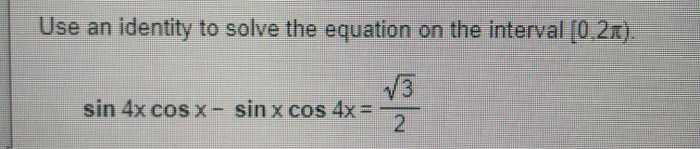Use an identity to solve the equation on the interval (0,21). 3 sin 4x cos x- sin x cos 4x = -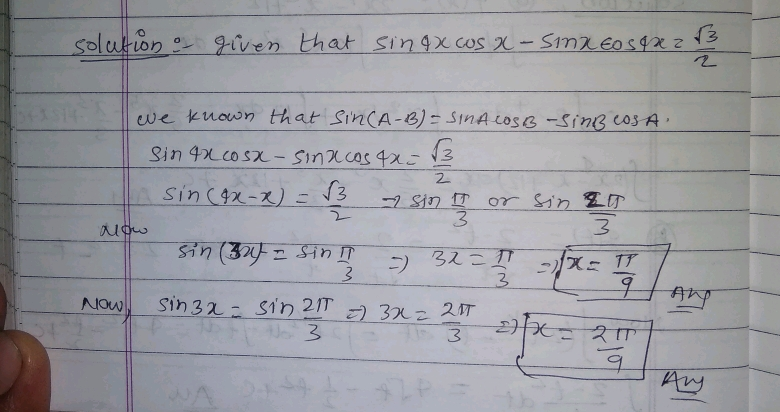#### Earn Coin

Coins can be redeemed for fabulous gifts.

Similar Homework Help Questions
• ### Use an identity to solve the equation on the interval [0,21). sin ?x-4 cos x +...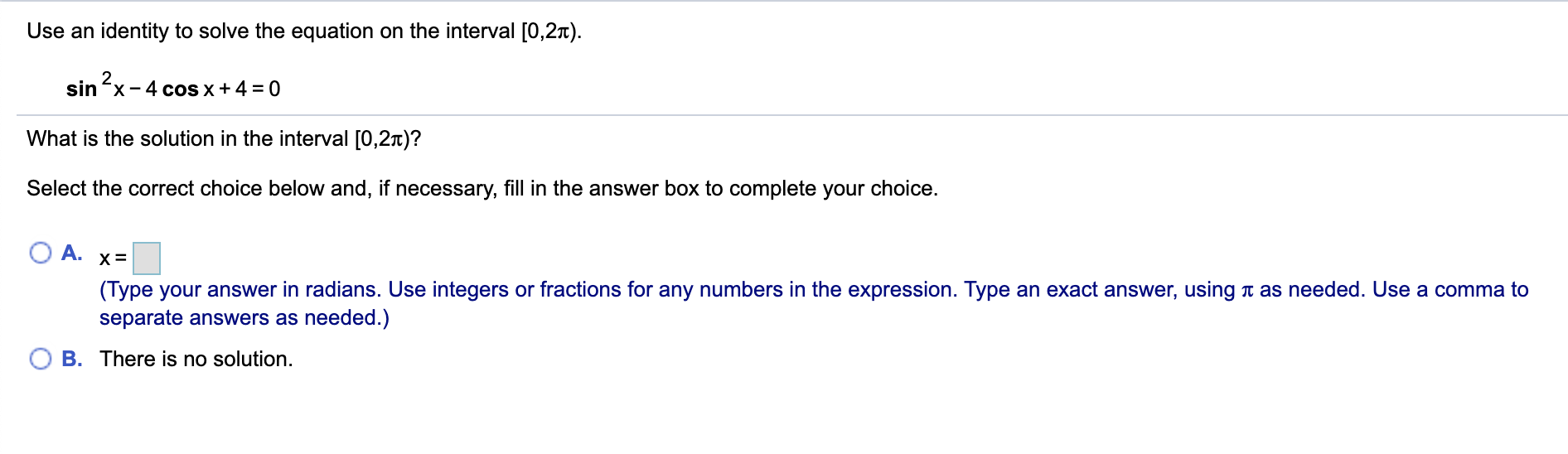Use an identity to solve the equation on the interval [0,21). sin ?x-4 cos x + 4 = 0 What is the solution in the interval [0,21)? Select the correct choice below and, if necessary, fill in the answer box to complete your choice. A. X= (Type your answer in radians. Use integers or fractions for any numbers in the expression. Type an exact answer, using a as needed. Use a comma to separate answers as needed.) B. There is...

• ### X 4.4.63 Use an identity to solve the equation on the interval (0,25). sin 4x cos...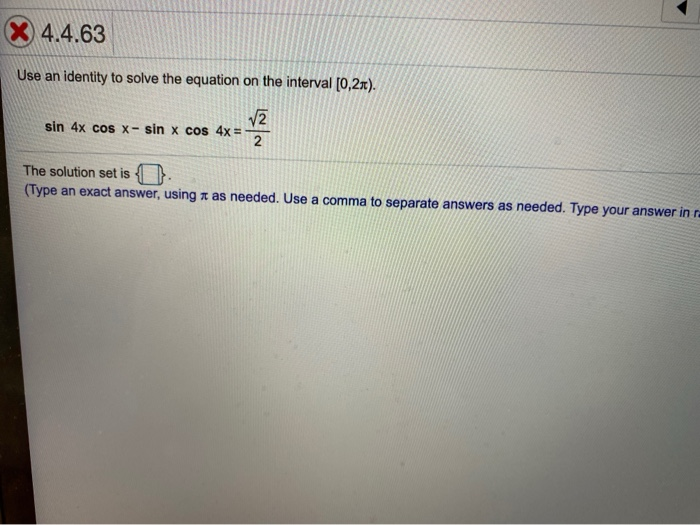X 4.4.63 Use an identity to solve the equation on the interval (0,25). sin 4x cos x- sin x cos 4x = v2 2 The solution set is O. (Type an exact answer, using as needed. Use a comma to separate answers as needed. Type your answer in

• ### Use an identity to solve the following equation on the interval [0,21). sin 2x = -12...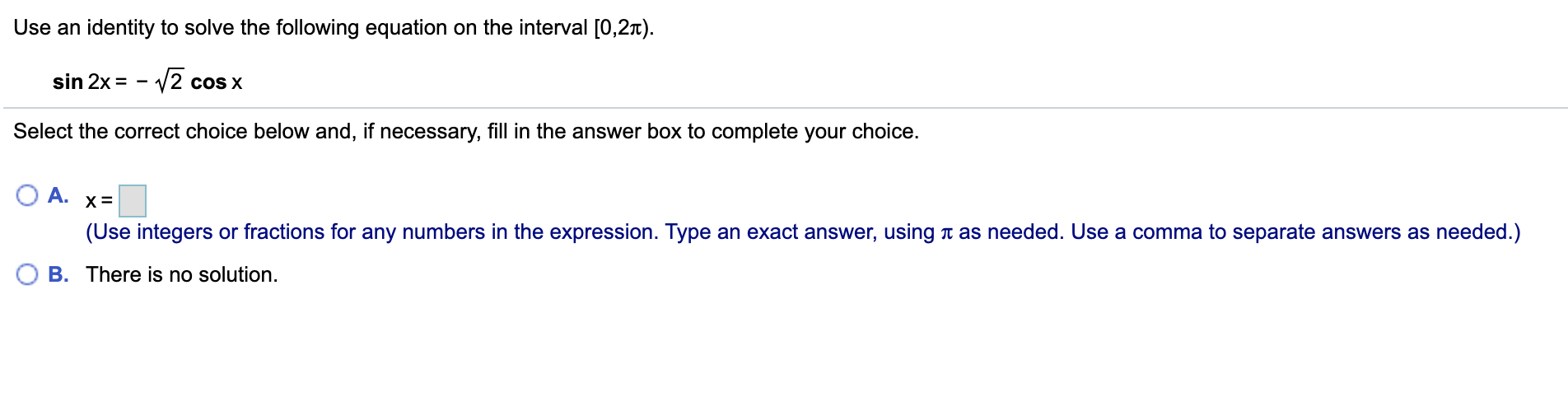Use an identity to solve the following equation on the interval [0,21). sin 2x = -12 cos x Select the correct choice below and, if necessary, fill in the answer box to complete your choice. O A. X= (Use integers or fractions for any numbers in the expression. Type an exact answer, using a as needed. Use a comma to separate answers as needed.) OB. There is no solution.

• ### cos2x+11cosx+6=0 Use an identity to solve the equation on the interval [0,21). cos 2x + 11...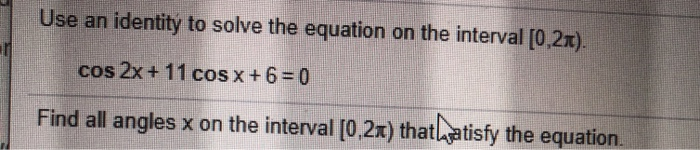cos2x+11cosx+6=0 Use an identity to solve the equation on the interval [0,21). cos 2x + 11 cos x + 6 = 0 Find all angles x on the interval [0,2x) thatlasatisfy the equation.

• ### Use an identity to solve the following equation on the interval [0,21). 2 cos2x- sin x-1=0...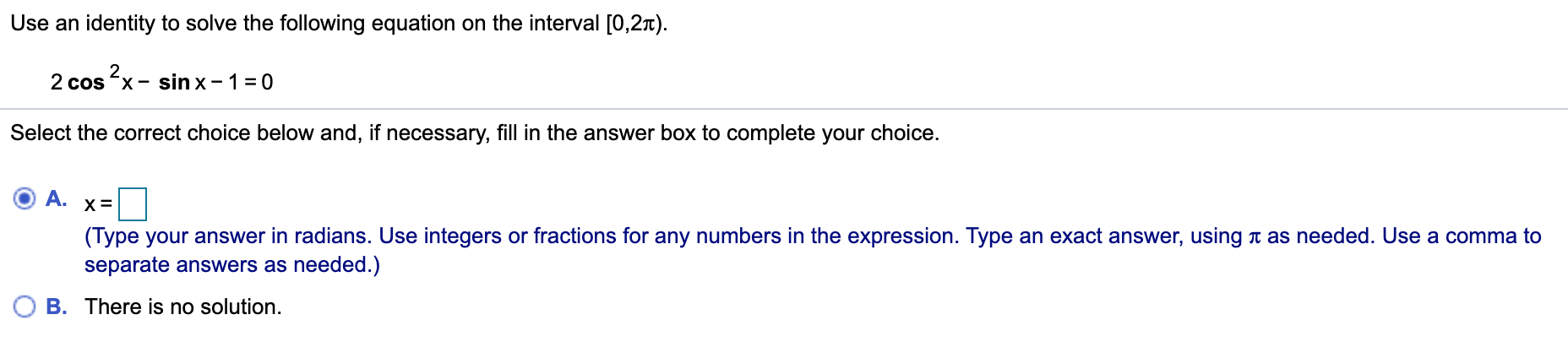Use an identity to solve the following equation on the interval [0,21). 2 cos2x- sin x-1=0 Select the correct choice below and, if necessary, fill in the answer box to complete your choice. A. X= (Type your answer in radians. Use integers or fractions for any numbers in the expression. Type an exact answer, using a as needed. Use a comma to separate answers as needed.) OB. There is no solution.

• ### Solve the equation on the interval [0.2π). (tan θ + 1)( cos θ-1):0 Use an identity to solve the following equation on the 2 2 cos x sin x 1 0 Select the correct choice below and, if necessa...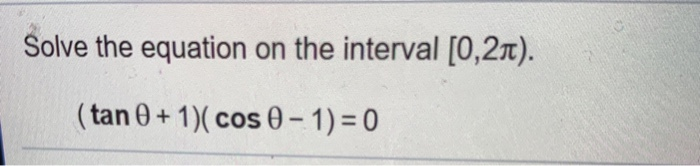Solve the equation on the interval [0.2π). (tan θ + 1)( cos θ-1):0 Use an identity to solve the following equation on the 2 2 cos x sin x 1 0 Select the correct choice below and, if necessary, filli (Type your answer in radians. Use integers ort separate answers as needed.) There is no solution. 0 B. Solve the equation on the interval [0.2π). (tan θ + 1)( cos θ-1):0 Use an identity to solve the following equation on...

• ### In Exercises 18-21, solve each trigonometric equation on the interval for [0,21). Use exact values for...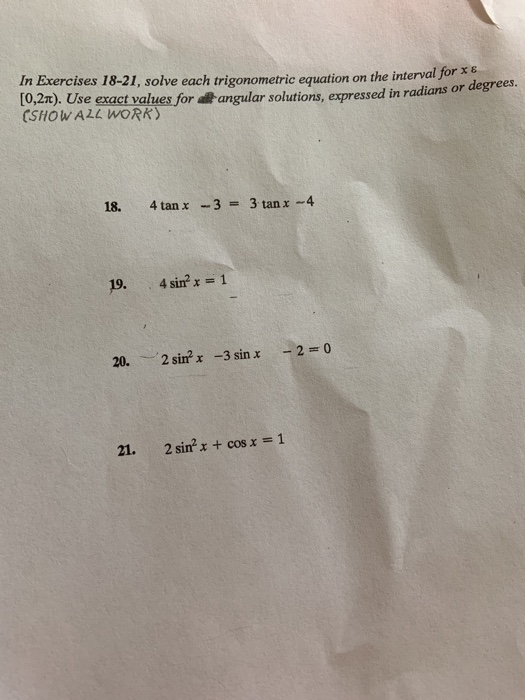In Exercises 18-21, solve each trigonometric equation on the interval for [0,21). Use exact values for at angular solutions, expressed in radians (SHOW ALL WORR) 18. 4 tan x - 3 = 3 tan x - 4 19. 4 sinº x = 1 20. 2 sinx -3 sin x - 2 = 0 21. 2 sin? + + cos x = 1

• ### Solve the equation on the interval [0,21). sin (20) = -1 What are the solutions to...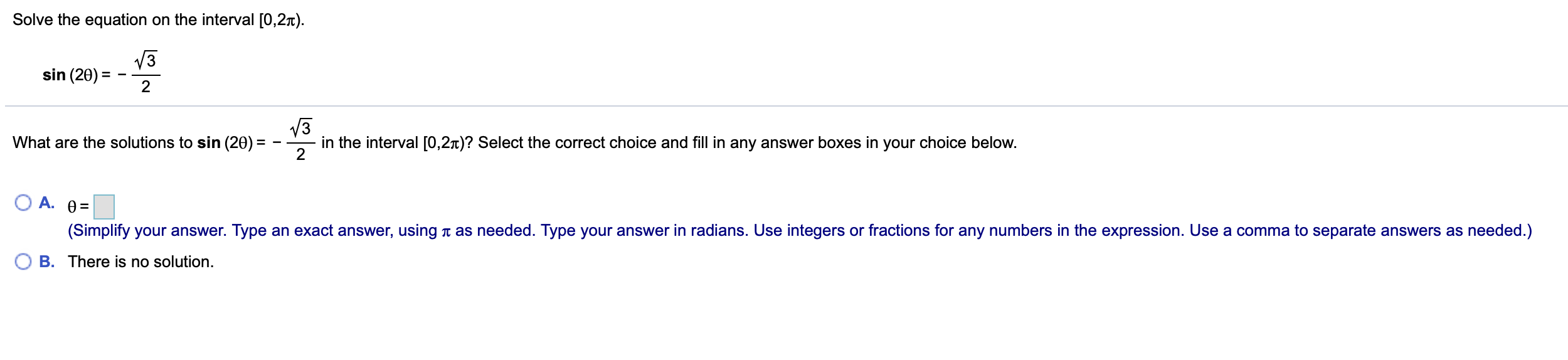Solve the equation on the interval [0,21). sin (20) = -1 What are the solutions to sin - in the interval [0,21)? Select the correct choice and fill in any answer boxes in your choice below. O A. 0= (Simplify your answer. Type an exact answer, using a as needed. Type your answer in radians. Use integers or fractions for any numbers in the expression. Use a comma to separate answers as needed.) OB. There is no solution.

• ### Verify the identity: sin 2x/sin x - cos 2x/cos x = sec x Solve the equation...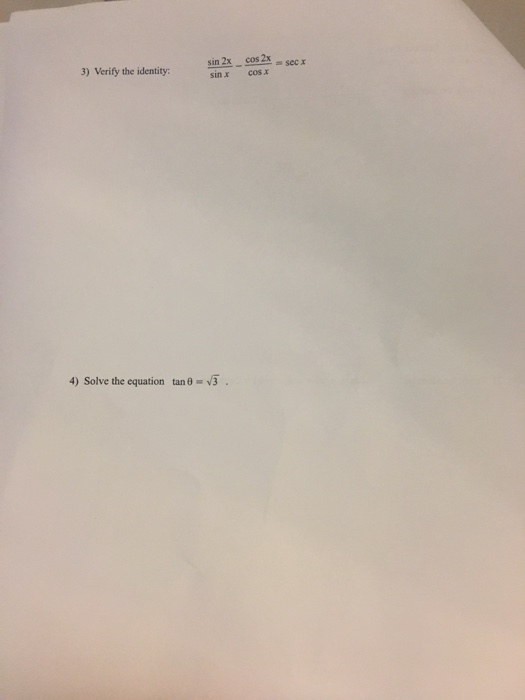Verify the identity: sin 2x/sin x - cos 2x/cos x = sec x Solve the equation tan theta = Squareroot 3.

• ### Use an appropriate identity to solve the given equation. 1 (a) sin(0) cos (35°) + cos(0)...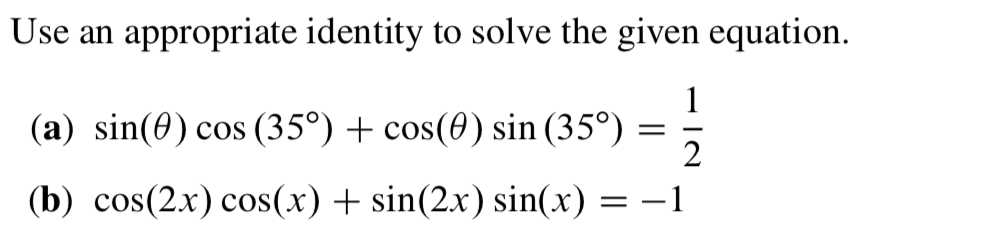Use an appropriate identity to solve the given equation. 1 (a) sin(0) cos (35°) + cos(0) sin (35°) = 2 (b) cos(2x) cos(x) + sin(2x) sin(x) = -1